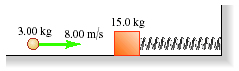# Problem: A 15.0 kg block is attached to a very light horizontal spring of force constant 475 N/m and is resting on a smooth horizontal table. (See the figure below .) Suddenly it is struck by a 3.00 kg stone traveling horizontally at 8.00 m/s to the right, whereupon the stone rebounds at 2.00 m/s horizontally to the left.Find the maximum distance that the block will compress the spring after the collision.(Hint: Break this problem into two parts - the collision and the behavior after the collision - and apply the appropriate conservation law to each part.)

###### FREE Expert Solution

The conservation of linear momentum equation:

$\overline{)\begin{array}{rcl}{\mathbf{m}}_{\mathbf{1}}{\mathbf{v}}_{\mathbf{01}}\mathbf{+}{\mathbf{m}}_{\mathbf{2}}{\mathbf{v}}_{\mathbf{02}}& {\mathbf{=}}& {\mathbf{m}}_{\mathbf{1}}{\mathbf{v}}_{\mathbf{f}\mathbf{1}}\mathbf{+}{\mathbf{m}}_{\mathbf{2}}{\mathbf{v}}_{\mathbf{f}\mathbf{2}}\end{array}}$

We'll also use the law of conservation of energy:

$\overline{){{\mathbf{K}}}_{{\mathbf{i}}}{\mathbf{+}}{{\mathbf{U}}}_{{\mathbf{i}}}{\mathbf{+}}{{\mathbf{W}}}_{{\mathbf{nc}}}{\mathbf{=}}{{\mathbf{K}}}_{{\mathbf{f}}}{\mathbf{+}}{{\mathbf{U}}}_{{\mathbf{f}}}}$, where Wnc is the work done by non-conservative forces such as friction.

For our case, Ui = 0 since there is no height (Remember U = mgh).

Wnc = 0 because there is no friction or any other non-conservative forces (we're told that the spring is on a smooth horizontal table).

After the collision, we do not have kinetic energy since the spring will be at rest.

86% (410 ratings)###### Problem Details

A 15.0 kg block is attached to a very light horizontal spring of force constant 475 N/m and is resting on a smooth horizontal table. (See the figure below.) Suddenly it is struck by a 3.00 kg stone traveling horizontally at 8.00 m/s to the right, whereupon the stone rebounds at 2.00 m/s horizontally to the left.

Find the maximum distance that the block will compress the spring after the collision.(Hint: Break this problem into two parts - the collision and the behavior after the collision - and apply the appropriate conservation law to each part.)

Frequently Asked Questions

What scientific concept do you need to know in order to solve this problem?

Our tutors have indicated that to solve this problem you will need to apply the Collisions with Springs concept. You can view video lessons to learn Collisions with Springs. Or if you need more Collisions with Springs practice, you can also practice Collisions with Springs practice problems.